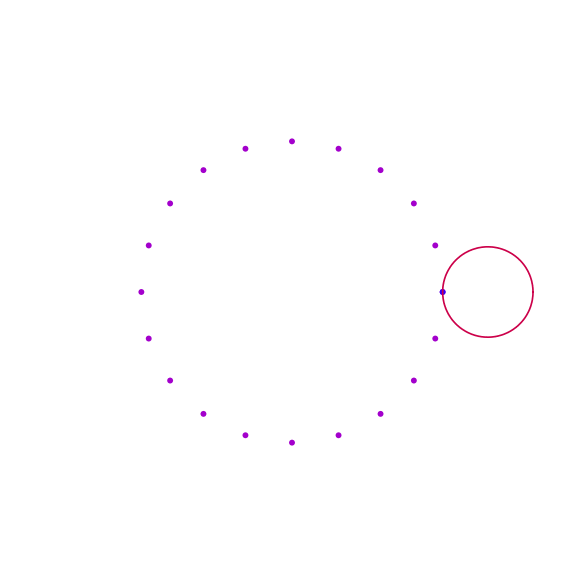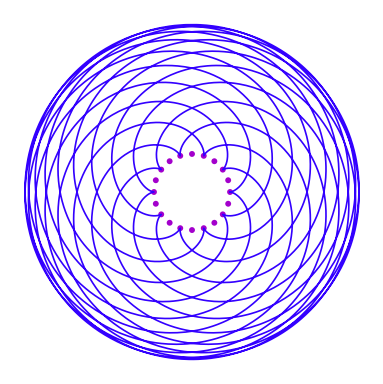# Fun Topics in Elementary Arithmetic

Here is a collection of fun topics more or less related to things we've done in class. Enjoy!

## Decimal digits of 1/B ¶

We learned in class that a rational number A/B has at most B-1 decimal digits before the decimal expansion begins to repeat. (We saw this by considering the remainder terms during long division.) But we also know that in many cases the decimal expansion begins to repeat before digit number (B-1). So for which values of B does the decimal expansion have the maximal number of non-repeating digits? To simplify the discussion, let's just start with the case A = 1:

• 1/3 = .3333...
• 1/6 = .16666...
• 1/7 = .142857 142857 ...
• 1/9 = .1111...
• 1/11 = .090909...
• 1/17 = .0588235294117647 0588235294117647 ...

So when B = 7 or 17, we have B-1 non-repeating digits, but in the other cases above we have fewer. Why does this happen?

When you apply long division, you'll see that the first remainder term (after 1) is the remainder term for 10 ÷ B. When you continue, note that the second remainder term winds up being the same as the remainder term for 100 ÷ B, and the third winds up being the same as the remainder term for 1000 ÷ B. Can you think of an explanation for why this happens?

It turns out that this does always happen: The remainder terms during long division for 1 ÷ B are the remainder terms for the successive powers of 10. The nth remainder term is the remainder of 10^n ÷ B.

Now this means that if you want 1/B to have B-1 non-repeating decimal digits, then you need the remainders of 10^n ÷ B to go through all the whole numbers from 1 to B-1. When this happens, 10 is said to be a primitive root modulo B. Primitive roots were studied by Euler and Gauss in the late 1700's, and Gauss wrote about them in his famous arithmetic book Disquisitiones Arithmeticae (1801) partly in order to understand decimal expansions of rational numbers, just as we're doing now!

Completely describing all primitive roots is still an unsolved problem, but we do know some things about them. For starters, some numbers don't have any primitive roots. The number B has a primitive root only in the following cases:

• B is a power of an odd prime,
• B is twice a power of an odd prime,
• B = 2, or
• B = 4.

The explanation for why these are the only cases is a little beyond what we've covered in class, but it would be covered in an introduction to number theory. When B does have primitive roots, it often has more than one, and 10 may or may not be among them. The primitive roots of B can't have any prime factors in common with B, so of the four cases when B can have primitive roots, the only one where 10 can be one of them is the first one: when B is a power of an odd prime. But even in this case 10 may or may not be a primitive root. For example, 10 is a primitive root for B = 7, 17, 19, 23, 29, 47, 49; but 10 is not a primitive root for B = 9, 11, 13, 25, 27, 31, 37, 41, 43. This means that 1/B will have B-1 non-repeating decimal digits when B is one of the numbers in the first list, but not when it is in the second list!

The question of which odd prime powers have 10 as a primitive root is an open question, and if you have any new ideas you should definitely let someone know!

## Spirograph-style flowers ¶

A spirograph-style flower made by rolling one circle around another. You can draw a similar shape with any number of dots in the center, and each petal traversing any number of dots.

Can you figure out how the total number of petals depends on these two numbers? Hint: try several examples, and consider the prime factorizations of the input numbers.Flower with 20 center dots and 6 dots per petal.

Here are some more gifs you might like:

The relationship between number of central dots, number of dots per petal, and total number of petals also works when the number of dots per petal is more than the number of central dots!Flower with 20 center dots and 34 dots per petal -- each petal winds around the center dots more than once. Click here for an animated gif drawing this design.

## The associagon ¶

Multiplication is associative, meaning that the two different ways of associating three numbers result in the same product

$(a \cdot b) \cdot c = a \cdot (b \cdot c).$

In higher-dimensional algebra, the associativity rule is replaced by a weaker rule. In that case, we need to think about the five ways to associate four numbers, and how the different ways are related.

For example, we have

$((a \cdot b) \cdot c) \cdot d \sim (a \cdot b) \cdot (c \cdot d)$

and

$(a \cdot b) \cdot (c \cdot d) \sim a \cdot (b \cdot (c \cdot d)).$

Can you find all of the ways to associate four numbers, and all of the relationships between them? They fit into a geometric shape called the associagon (it also goes by a different name that you probably already know). There are higher-dimensional analogs of this for associating five or more numbers, starting with the associahedron!

tags: fun-topics

## Site InfoThe content of nilesjohnson.net is licensed under CC BY-NC 4.0.